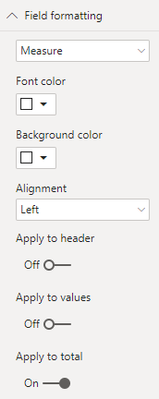cancel
Showing results for
Did you mean:Helper II

## Hide Columun Measure Total

Dear All,

I have a measure dividing two measure by each other -

Measure 2 = [Measure 5]/[Measure 6])

Is there a way to hide the total for this Measure 2 & Measure 3. The total is not correct.I need to remove the circle ones.

Thanks.

Regards

1 ACCEPTED SOLUTIONFrequent Visitor

You are looking for the opposite of this question https://community.powerbi.com/t5/Desktop/Measure-does-not-show-total/td-p/514010

The same logic applies. A workaround would be to change the formula to include the level of detail you are displaying. So if you want to calculate the Measure for customers but not for the total, you could do something like this:

`Measure = if(HASONEVALUE('Your table'[Your Customer Field]);[Measure 1]/[Measure 2];Blank())`

Note the empty value in the Measure for the total row (Totaal).The Customer Field would now have to be in the visual for the Measure to display a value. So if you want to perform the same calculation based on regio, you would have to add another measure.

2 REPLIES 2Frequent Visitor

You are looking for the opposite of this question https://community.powerbi.com/t5/Desktop/Measure-does-not-show-total/td-p/514010

The same logic applies. A workaround would be to change the formula to include the level of detail you are displaying. So if you want to calculate the Measure for customers but not for the total, you could do something like this:

`Measure = if(HASONEVALUE('Your table'[Your Customer Field]);[Measure 1]/[Measure 2];Blank())`

Note the empty value in the Measure for the total row (Totaal).The Customer Field would now have to be in the visual for the Measure to display a value. So if you want to perform the same calculation based on regio, you would have to add another measure.Solution Sage

Hi,

One thing you could try is to hide the total by changing the font colors to the same as the background for the measure. This can be done under field formatting and apply it just to total.